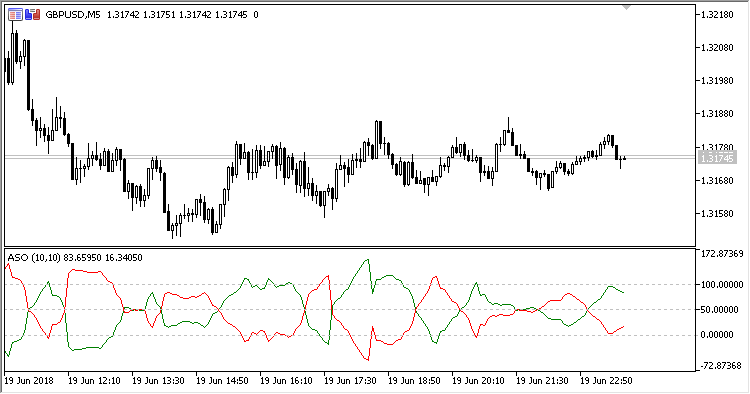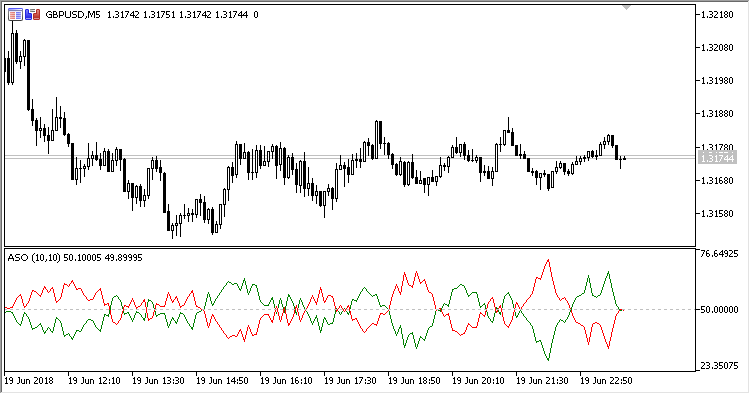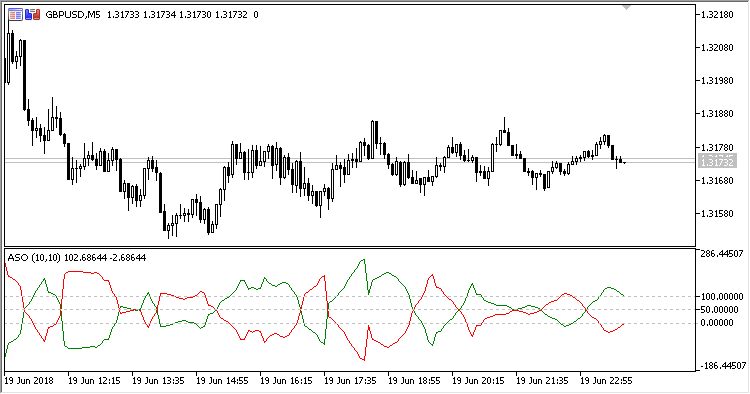• Get access

Interesting script?
So post a link to it -
let others appraise it

You liked the script? Try it in the MetaTrader 5 terminal# ASO - indicator for MetaTrader 5

Views:
1752
Rating:
Published:
2018.07.13 15:19

Indicator ASO (Average Sentiment Oscillator) displays the general market climate.

It has four configurable parameters:

• Range period - range calculation period;
• Smoothing period - oscillator smoothing period;
• Method - smoothing method;
• Mode - calculation mode:
• Combined - combined mode;
• Intra-bar - intra-bar mode;
• Group algorithm - group algorithm mode.

Calculations:

```Bulls = MA(BL, Smoothing period, Method)
Bears = MA(BR, Smoothing period, Method)
```

where:

Combined Mode:

```BL = (IBL+GBL)/2
BR = (IBR+GBR)/2
```

Intra-bar Mode:

```BL = IBL
BR = IBR
```

Group Algorithm Mode:

```BL = GBL
BR = GBR
IBL = 50 * (Close - Low + High - Open)/(High - Low)
IBR = 50 * (High - Close + Open - Low)/(High - Low)
GBL = 50*(Close - GL + GH - GO)/(GH - GL)
GBR = 50*(GH - Close + GO - GL)/(GH - GL)
```
• GO - Open within Range period;
• GL -minimum value of High within Range period;
• GH - maximum value of Low within Range period.Fig 1. Combined ModeFig. 2. Intra-bar ModeFig. 2. Group Algorithm Mode

Translated from Russian by MetaQuotes Software Corp.
Original code: https://www.mql5.com/ru/code/21159SymbolX_Candle

This indicator calculates the index of a given currency, using the USD index. It is based on indicator SymbolX, but four prices are used for calculations: OHLC instead of just one Close price.SymbolX

This indicator calculates the index of a given currency, using the USD index. The Close prices of six currency pairs are used to calculate the index.CA

Indicator CA (Corrected Average), also known as Optimal Moving Average. The benefit of the indicator is the fact that the current value of the timeseries must exceed the current threshold that depends on volatility for the indicator line to follow the price, avoiding false signals in the trend.DMX

Indicator DMX (Bipolar DMI) is calculated using the standard indicator ADX. However, as compared to that, it displays data as an oscillator with a signal line, and has shorter delay.